Courses

# HC Verma Test: Current Electricity

## 30 Questions MCQ Test Physics Class 12 | HC Verma Test: Current Electricity

Description
This mock test of HC Verma Test: Current Electricity for Class 12 helps you for every Class 12 entrance exam. This contains 30 Multiple Choice Questions for Class 12 HC Verma Test: Current Electricity (mcq) to study with solutions a complete question bank. The solved questions answers in this HC Verma Test: Current Electricity quiz give you a good mix of easy questions and tough questions. Class 12 students definitely take this HC Verma Test: Current Electricity exercise for a better result in the exam. You can find other HC Verma Test: Current Electricity extra questions, long questions & short questions for Class 12 on EduRev as well by searching above.
QUESTION: 1

### The electric current in a liquid is due to the flow of

Solution:

In liquids the current is carried by ions. Ions are charged particles (atoms or groups of atoms). Ions can either be positive or negative, the positive ions being attracted to the negative plate and the negative ions to the positive plate. A positive ion is a particle with some negative charge taken away and a negative ion is a particle with some extra negative charge added.

QUESTION: 2

### The electric current in a discharge tube containing a gas is due to

Solution:

Thus, intensive recombinations of electrons and positive ions into neutral atoms and molecules occur. This results in the emission of light by the discharge, i.e., the negative glow is mainly due to a glow of recombination. Hence in electric discharge the electric current is due to both electrons and positive ions.

QUESTION: 3

### A current (I) flows through a uniform wire of diameter (d) when the mean drift velocity is V. The same current will flow through a wire of diameter d/2 made of the same material if the mean drift velocity of the electron is

Solution: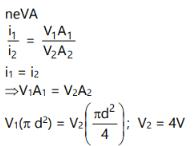QUESTION: 4

When a potential difference (V) is applied across a conductor , the thermal speed of electrons is

Solution:
QUESTION: 5

A steady current is passing through a linear conductor of non-uniform cross-section. The current density in the conductor is

Solution:

Current density is equal to Electric current divided by a given surface area, or it is the current flowing per a given cross section area.
The current density decreases with increase in cross section area which means they are inversely proportional.

QUESTION: 6

A metallic block has no potential difference applied across it. Then the mean velocity of free electron is

Solution:

When there is no Potential Difference across the block, the motion of electrons will not be systematic. They will move in random motion.
By considering the motion of all the free electron in every possible direction due to randomness, the Average mean velocity of free electrons turns out to be zero.

QUESTION: 7

The resistance of some substances become zero at very low temperature , then these substances are called

Solution:

Superconductivity is a phenomenon of exactly zero electrical resistance and expulsion of magnetic fields occurring in certain materials when cooled below a characteristic critical temperature.
Even near absolute zero, a real sample of a normal conductor shows some resistance. In a superconductor, the resistance drops abruptly to zero when the material is cooled below its critical temperature. An electric current flowing through a loop of superconducting wire can persist indefinitely with no power source.
Example: - Liquid Nitrogen.

QUESTION: 8

The resistance of wire is 20W. The wire is stretched to three times its length. Then the resistance will now be

Solution:

Unit of resistance should be 20ohm

Solution :- Resistance, R= ρl/A

When length l increases three times, the cross section A will decease three times of previous. So length becomes 3l and cross section becomes A/3.

Now resistance becomes ,R  = ρ3l / πA/3

​ =9R=9×20=180Ω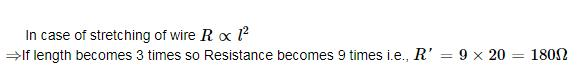QUESTION: 9

The resistance of a semi-conductors

Solution:

The resistivity of a semiconductor decreases with temperature. This is because of increasing temperature, the electrons in the valence band gain sufficient thermal energies to jump to the conduction band. As the number of electrons in the conduction band increases, so conductivity increases and resistivity decreases.

QUESTION: 10

A hollow copper tube of 5 m length has got external diameter equal to 10 cm and the walls are 5 mm thick. If specific resistance of copper is 1.7 × 10-8 ohm × metre. calculate the resistance of the tube.

Solution:

Cross sectional area of the copper tube is:
A=(π/4)​(d12​−d22​)=(π/4)​(102−92)=14.9cm2=14.9∗10−4m2
l=5m
ρ=1.7∗10−8
Resistance is given by: R=ρl/A​=5.77 x 10−5Ohm

QUESTION: 11

In order to increase the resistance of a given wire of uniform cross section to four times its value, a fraction of its length is stretched uniformly till the full length of the wire becomes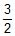times the original length what is the value of this fraction ?

Solution:

Let, the length of wire = l unit

Fraction required =a

Then, the lenght of wire which is stretched =al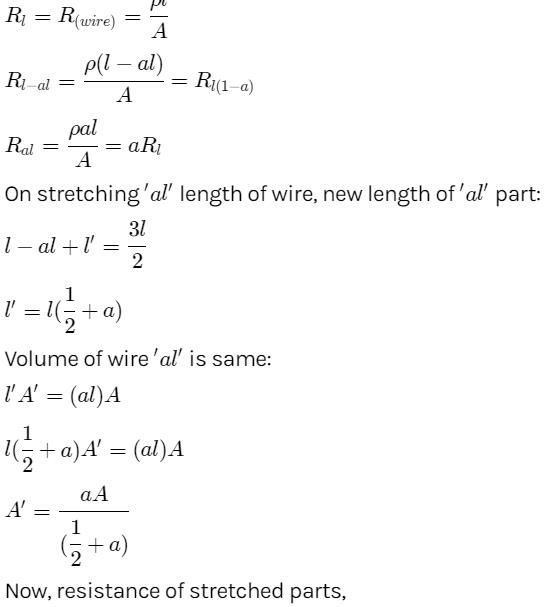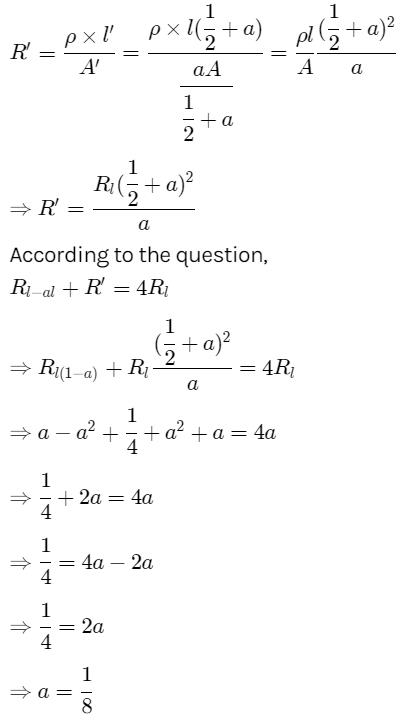QUESTION: 12

A conductor with rectangular cross section has dimensions (a × 2a × 4a) as shown in figure. Resistance across AB is x, across CD is y and across EF is z. Then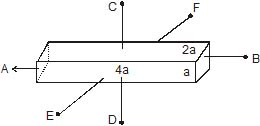Solution:

Resistance of a wire of length l and cross-sectional area A is given by R=ρl/A​
here, x=ρ(4a)​/(2a×a)= 2ρ/a​ ;
y=ρ(a)​/ 2a×4a=ρ/8a​ and
z= ρ(2a)/4a×a​=ρ/2a​
thus, x>z>y

QUESTION: 13

A negligibly small current is passed through a wire of length 15 m and uniform cross-section 6.0×10-7 m2. The measured resistance of the wire is 5.0 W. The resistivity of the material is

Solution:

Length of the wire, l =15 m
Area of cross-section of the wire, a = 6.0 × 10−7 m2
Resistance of the material of the wire, R = 5.0 Ω
Resistivity of the material of the wire = ρ
Resistance is related with the resistivity as,
ρ=RA/l
=5.0×6×10−7/15
=0.2×10−6Ωm

QUESTION: 14

The resistance of a wire of length 20 cm is 5W. It is stretched uniformly to a length of 40 cm. The resistance now becomes:

Solution:

Unit of resistance should be 5ohm.

Resistance of a wire is given by the formula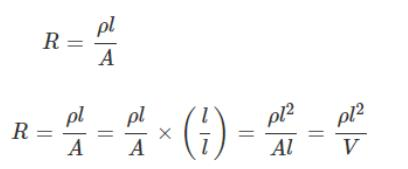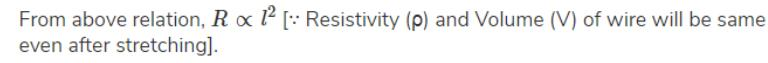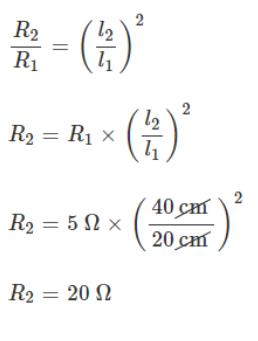QUESTION: 15

Two wires each of radius of cross section r but of different materials are connected together end to end (in series). If the densities of charge carriers in the two wires are in the ratio 1 : 4, the drift velocity of electrons in the two wires will be in the ratio.

Solution:

Let  n1​ and n2​ be the densities of charge carriers in the two wires, Vd1​​ and Vd2​​ be the drift velocities of charge carriers in the two wires,
A be the area of cross section,
e is the charge on electron and
I is the current flowing through both the wires
Hence, I=n1​eAVd1​​=n1​eAVd2​​
∴n1​Vd1​​=n2​Vd2​​
∴ Vd1/Vd2​​ ​​​=​n2​​/n1
∴ ​​Vd1​​​/ Vd2=4/1​

QUESTION: 16

In a wire of cross-section radius r, free electrons travel with drift velocity v when a current I flows through the wire. What is the current in another wire of half the radius and of the same material when the drift velocity is 2v ?

Solution:

Here we are using the formula
current = neAv ...............(1)
where n= number of electron density
A =area of cross section
e= charge of electron
v=drift velocity
As both the wires are of the same materials then the electron density is the same for both.
For the first wire, current I is given by,
I = neAv
Now according to the problem another wire of half the radius and of the same material when the drift velocity is 2v
Therefore for the second wire current is = ne(A/4)(2v)
= (neAv)/2
now putting I at this place
= I/2

QUESTION: 17

Read the following statements carefully :

Y : The resistivity of a semiconductor decreases with increases of temperature.

Z : In a conducting solid, the rate of collision between free electrons and ions increases with increase of temperature.

Select the correct statement from the following

Solution:

In case of semiconductor Resistivity∝1​/temperature
In this case as temperature increases electrons jump to conduction band resistance decreases R∝ρ(Resistivity) so resistivity also decreases and current increases.
Vibration of electrons increases with rise in temperature and hence collision between ions and electrons increases with temperature.

QUESTION: 18

A piece of copper and another of germanium are cooled from room temperature to 80K. The resistance of

Solution:

We know, a piece of copper is a metal while that of germanium is a semiconducting material. For metals resistance increases with increase in temperature. The semiconductor has a negative temperature coefficient of resistance. Hence, when it is cooled its resistance increases.

QUESTION: 19

An insulating pipe of cross-section area `A' contains an electrolyte which has two types of ions ® their charges being _e and +2e. A potential difference applied between the ends of the pipe result in the drifting of the two types of ions, having drift speed = v (_ve ion) and v/4 (+ ve ion). Both ions have the same number per unit volume = n. The current flowing through the pipe is

Solution:

I1 = n x -(e) x A x V
I2 = n x 2e x A x V/4
= n x e x A x V/2
Total Current = I1 + I2
=nea(V/2) - (-neav) (Since both ions will move in opposite directions,  we subtract them)
Hence Current I= (neav/2) + neav
= 3/2neaV

QUESTION: 20

Two wires of the same material having radii in the ratio 1:2, carry currents in the ratio 4 : 1. The ratio of drift velocities of electrons in them is

Solution:

We have the relation between current I, n = no. of carrier per unit volume, drift velocity ve and CSA A as.

I = neAv

Let current flowing in the 2nd wire I2 = I thus current flowing through the 1st wire I1 = 4I

Let the radius of the first wire be r thus radius of the 2nd wire = 2r

In the first wire

A1 = πr2

In the 2nd wire

A2 = π(2r)2 = 4πr2

I1/ I2 = (A1v1 )/(A2v2 )  (ne being same for two wires as they are made of the same material)

4I/I = πr2 v1/4 πr2v2

=> v1/v2 = 16/1

=> v­1:v2 = 16:1

Thus ratio of the drift velocities are 16:1

QUESTION: 21

A current I flows through a uniform wire of diameter d when the mean electron drift velocity is v. the same current will flow through a wire of diameter d/2 made of the same material if the mean drift velocity of the electron is

Solution: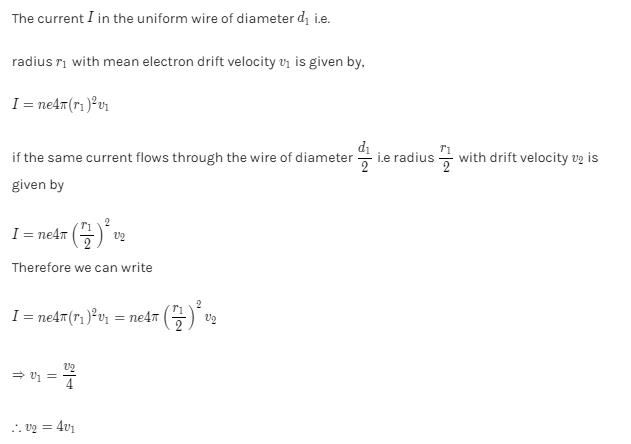QUESTION: 22

The current in a metallic conductor is plotted against voltage at two different temperatures T1 and T2. Which is correct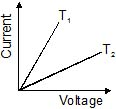Solution:

According to Ohm's law R=V/I​
Where R= resistance, V= Voltage, I= Current
Also R  is directly proportional to temperature T and inversely proportional to the slope.
∴ from the above example
R1​∝T1​ and R2​∝T2
but from given figure R1​<R2
∴T1​<T2

QUESTION: 23

A uniform copper wire carries a current i amperes and has p carriers per metre3. The length of the wire is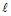metres and its cross-section area is s metre2. If the charge on a carrier is q coulombs, the drift velocity in ms-1 is given by

Solution:

The current flowing through the wire is given by
I=neAv
Where I = current
n = charge carrier current density
e = charge on the carriers
A = cross sectional area
v = drift velocity
In the above example
I = current = i
n = charge carrier current density = p
e = charge on the carriers = q
A = cross sectional area = s
v = drift velocity = v
hence i=pqsv
∴v=i/pqs

QUESTION: 24

In the presence of an applied electric field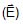in a metallic conductor.

Solution:

Conduction of electrons in a conductor.
The motion of electrons is random but in presence of external electric field, they slowly drift opposite to  and cause a current

QUESTION: 25

A wire has a non-uniform cross-section as shown in figure. A steady current flows through it. The drift speed of electrons at points P and q is vp and vQ.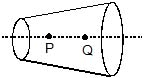Solution:

The drift speed of electrons is inversely proportional to the radius of cross section.
Thus here the cross-sectional area at point P is smaller than the cross-sectional area at point Q. Hence, the drift velocity is large for smaller area and small for larger area. The drift speed will relate as per the relation given below
vP​>vQ​

QUESTION: 26

A rectangular carbon block has dimensions 1.0 cm × 1.0 cm × 50 cm. Resistances are measured, first across two square ends and then across two rectangular ends, respectively. If resistivity of carbon is 3.5 × 10_5 W-m, then values of measured resistances respectively are :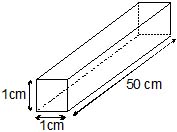Solution:

Between two square faces the area is 0.01×0.01=1×10−4m2 and length is 0.5m. So, the resistance is (3.5×10−5×0.5)/(1×10-4)=0.175ohm, this can be written as, (35/2) x10-2 ohm
Between the rectangular face the area is 0.01×0.5=0.005m2 and the length is 0.01m, hence the resistance is (3.5×10−5×0.01)/0.005=7×10-5 ohm

QUESTION: 27

A storage battery is connected to a charger for charging with a voltage of 12.5 Volts. The internal resistance of the storage battery is 1 W. When the charging current is 0.5 A, the emf of the storage battery is :

Solution:

Given A storage battery is connected to a charger for
charging with a voltage of 12.5 Volts. The internal
resistance of the storage battery is 1 ohm. When the
charging current is 0.5 A, the emf of the storage
battery is:
When the battery is charging
We know that
V = e + i r
V = 12.5, i = 0.5, r = 1
12.5 = e + 0.5 x 1
12.5 = e + 0.5
e = 12.5 – 0.5
e = 12 v

QUESTION: 28

The terminal voltage across a battery of emf E can be

Solution:

Potential difference(V) across the terminals of a cell
i. Discharging of cell when cell is supplying current,
Therefore, V=VP+VQ=E-Ir, i,r., V<E
ii. charging of cell when cell is taking current,
V=VP-VQ=E+Ir, i.e., V>E
iii. When the cell is short circuited i.e., external resistance is zero,
=> I=E/r
=>  V=VP-VQ=E-Ir=0 i.e., V=0

QUESTION: 29

In order to determine the e.m.f of a storage battery it was connected in series with a standard cell in a certain circuit and a current I1 was obtained. When the battery is connected to the same circuit opposite to the standard cell a current I2 flow in the external circuit from the positive pole of the storage battery was obtained. What is the e.m.f e1 of the storage battery? The e.m.f of the standard cell is e2.

Solution:

ε12=I1R,ε1−ε2=I2R
Dividing ε1=( I1+I2/I1-I22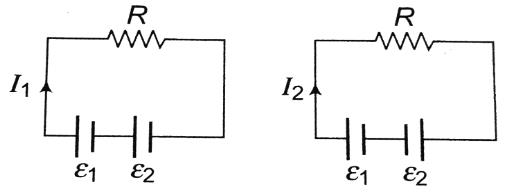QUESTION: 30

One end of a Nichrome wire of length 2L and cross-sectional area A is attatched to an end of another Nichrome wire of length L and cross-sectional area 2A. If the free end of the longer wire is at an electric potential of 8.0 volts, and the free end of the shorter wire is at an electric potential of 1.0 volt, the potential at the junction of the two wires is equal to

Solution: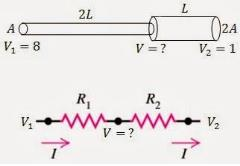Let V1​, I1​ be the voltage and current for Nichrome wire of length 2L and cross-sectional area A.
V2​,I2​ be the voltage and current for Nichrome wire of length L and cross-sectional area 2A.
According to Kirchhoff's law,
I1​= ​(V1​−V)​/R1
I2​=​(V−V2​)​/R2
where R is thee resistance of Nichrome wire,
R=ρl/A​
Hence,
R1​=ρ2L/A​=2R
R2​= ρL/2A ​=0.5R
Since current entering from one end leaves from another end,
I1​=I2​
(8−V​)/2=(V−1)/0.5​
V=6/2.5​=2.4V